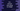# Different ways to convert one string to integer in C++# String to integer conversion in C++:

We have a couple of different ways to convert one string to int in C++. In this post, we will learn how to do this conversion with different examples.

## Using atoi :

This method is defined as below :

``int atoi(const char *str);``

This method takes one c string or char* and returns its integer equivalent. For example :

``````#include <iostream>
#include <cstdlib>
using namespace std;

int main()
{
string strNumberArray[] = {"1", "99", "999", "9999","-22", "0.1", "1.23", "-1.2", "1234.5678"};
int no;

for (string str : strNumberArray)
{
no = atoi(str.c_str());
cout << "Value of : " << str << " is : " << no << endl;
}
}``````

It will print the below output :

``````Value of : 1 is : 1
Value of : 99 is : 99
Value of : 999 is : 999
Value of : 9999 is : 9999
Value of : -22 is : -22
Value of : 0.1 is : 0
Value of : 1.23 is : 1
Value of : -1.2 is : -1
Value of : 1234.5678 is : 1234``````

In this example, we have one string array. Using one for loop, we are iterating through the elements of this array and printing out the integer value of each value.

Note that it only considers the integral part of the number.

## Using stoi :

stoi is similar to atoi. stoi was added in C++ 11. The main difference between atoi and stoi is that atoi works only with C style string but stoi works with both c style string and C++ string. We are using the same above string array here. Similar to atoi, stoi also considers only the integral part of the number.

For example :

``````#include <iostream>
#include <cstdlib>
using namespace std;

int main()
{
string strNumberArray[] = {"1", "99", "999", "9999","-22", "0.1", "1.23", "-1.2", "1234.5678"};
int no;

for (string str : strNumberArray)
{
no = stoi(str);
cout << "Value of : " << str << " is : " << no << endl;
}
}``````

It will print the below output :

``````Value of : 1 is : 1
Value of : 99 is : 99
Value of : 999 is : 999
Value of : 9999 is : 9999
Value of : -22 is : -22
Value of : 0.1 is : 0
Value of : 1.23 is : 1
Value of : -1.2 is : -1
Value of : 1234.5678 is : 1234``````

## Using sscanf :

sscanf takes three parameters: the first one is a c type string, the second one is the format type and the third one is the variable to store the result. It reads the content of the string, formats it using the format type and stores that value in the third parameter variable. We can use sscanf to convert one string to an integer. The second parameter will be %d for this conversion :

``````#include <iostream>
using namespace std;

int main()
{
string strNumberArray[] = {"1", "99", "999", "9999","-22", "0.1", "1.23", "-1.2", "1234.5678"};
int no;

for (string str : strNumberArray)
{
sscanf(str.c_str(), "%d", &no);
cout << "Value of : " << str << " is : " << no << endl;
}
}``````

Output :

``````Value of : 1 is : 1
Value of : 99 is : 99
Value of : 999 is : 999
Value of : 9999 is : 9999
Value of : -22 is : -22
Value of : 0.1 is : 0
Value of : 1.23 is : 1
Value of : -1.2 is : -1
Value of : 1234.5678 is : 1234``````

Only the integral part is considered.

## Using stringstream :

Using stringstream, we can read the contents of a string and output the content to an integer. stringstream.str() method is used to read the string content.

``````#include <iostream>
#include <sstream>
using namespace std;

int main()
{
string strNumberArray[] = {"1", "99", "999", "9999","-22", "0.1", "1.23", "-1.2", "1234.5678"};
int no;
stringstream stream;

for (string str : strNumberArray)
{
stream.clear();
stream.str(str);
stream >> no;
cout << "Value of : " << str << " is : " << no << endl;
}
}``````

output :

``````Value of : 1 is : 1
Value of : 99 is : 99
Value of : 999 is : 999
Value of : 9999 is : 9999
Value of : 0.1 is : 0
Value of : 1.23 is : 1
Value of : -1.2 is : -1
Value of : 1234.5678 is : 1234``````

It returns 0 if the value can’t be parsed.